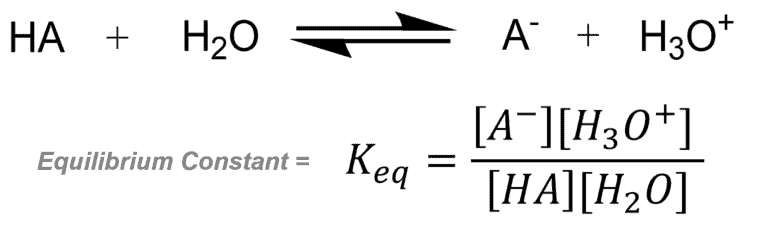# Equilibrium

## Equilibrium Definition:

Equilibrium is the stage reached in a reaction when there is no observable change in the concentration of the reactants and products.

## Equilibrium Explained:

For reversible reactions, saying that a reaction has reached equilibrium, means that the rate fo the forward reaction is equal to the rate of the reverse reaction and the reaction reaches a stable state. We must not confuse equilibrium as a point when the reaction ‘stops’. Hence, even under equilibrium, the reaction does take place, only such that, the rate at which the products are formed is equal to the rate at which the products dissociate and there is no net accumulation on wither side of the reaction.

In other cases, when the reactants react completely to form the products i .e irreversible reactions, equilibrium is the stage when no further change in concentration is seen.

Hence, Equilibrium = No ‘Rate of change’ in reactants and products.

Understanding Equilibrium

Consider an example – Acid-Base ReactionThe figure below shows how the rates become equal.The figure below shows how the concentrations are no longer changing.